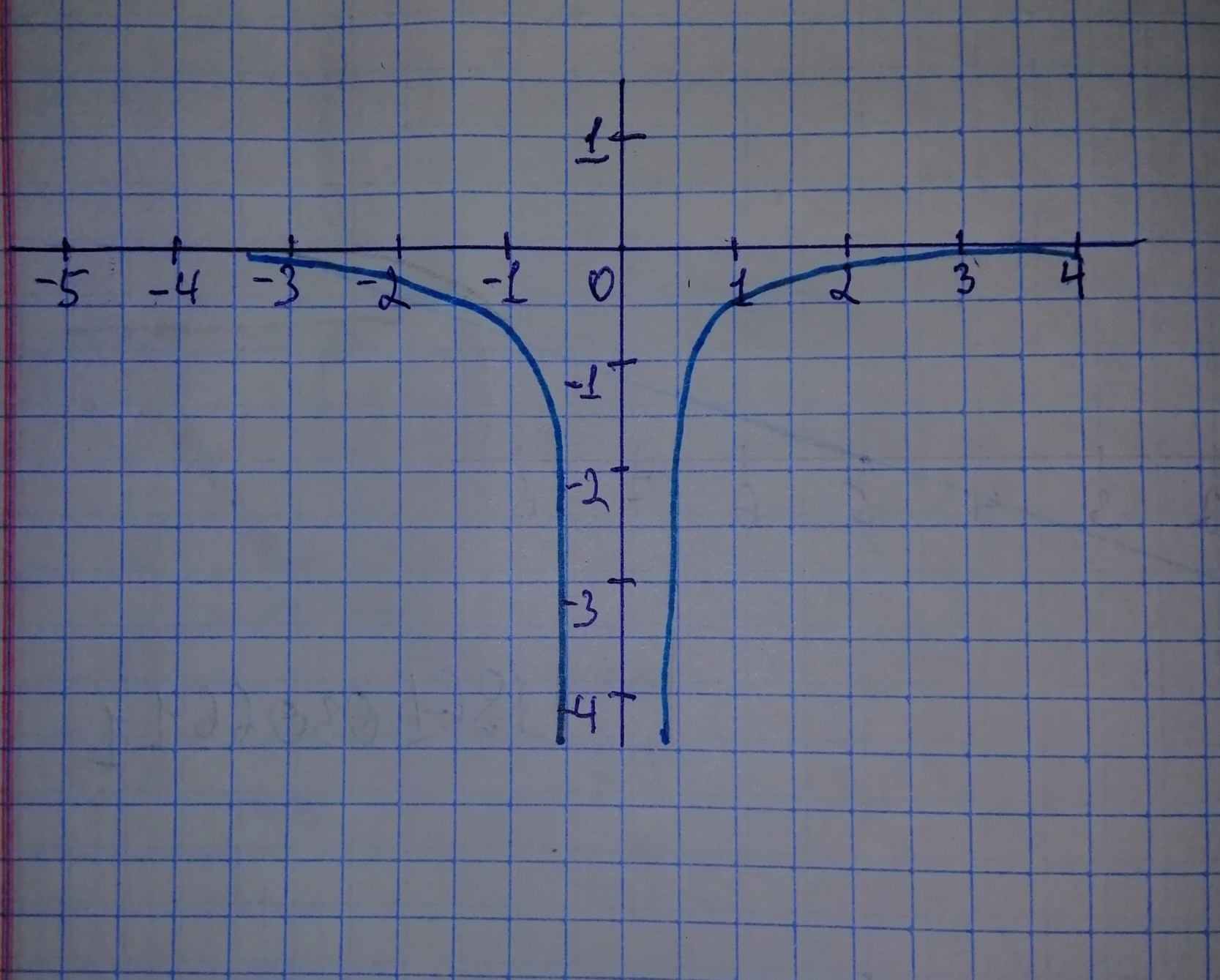Question# Sketch a graph of the function. Use transformations of functions when ever possible. f(x)= -frac{1}{x^{2}}

Transformations of functions
ANSWEREDSketch a graph of the function. Use transformations of functions when ever possible. $$\displaystyle{f{{\left({x}\right)}}}=\ -{\frac{{{1}}}{{{x}^{{{2}}}}}}$$Shown is graph of $$\displaystyle{f{{\left({x}\right)}}}=\ -{\frac{{{1}}}{{{x}^{{{2}}}}}}$$ x is on the horizontal axis and $$\displaystyle{y}={f{{\left({x}\right)}}}$$ is on the vertical axis.